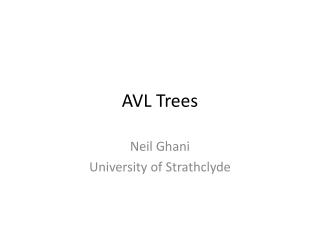DownloadDownload PresentationAVL Trees

# AVL Trees

Télécharger la présentation## AVL Trees

- - - - - - - - - - - - - - - - - - - - - - - - - - - E N D - - - - - - - - - - - - - - - - - - - - - - - - - - -
##### Presentation Transcript

1. AVL Trees Neil Ghani University of Strathclyde

2. General Trees • Recall a tree is * A leaf storing an integer * A node storing a left subtree, an integer and a right subtree. • We define algorithms by saying what they do to leaves and what they do to nodes

3. Example – searching a tree • For example, we can search a tree for data find z (leaf x) = (x == z) find z (node l x r) = find z l or (z == x) or find z r • This is clearly a O(n) algorithm. Can we do better and find a logarithmic algorithm

4. Binary Search Trees • With ordered lists we can search in logarithmic time. An ordered tree is called a binary search tree (BST) • A tree of the form leaf x is a BST • A tree of the form (node l x r) is a BST if * l and r are BSTs * max l <= x * min r >= x

5. Searching a BST • Searching a BST can use the fact that a BST is ordered findBST z (leaf x) = (x == z) findBST z (node l x r) = if (z == x) then true else if (z <= x) then findBST z l else findBST z r

6. Complexity of findBST • There is one recursive call * If the left and right subtrees are about the same size, T(n) = 2 + T(n/2) * If the right subtree is a leaf and we search the left subtree, T(n) = 2 + T(n-2) • Worst case complexity is linear

7. AVL Trees • AVL trees ensure that the left and right subtrees are about equal size and hence allow logarithmic searching. • Before talking about AVL trees, note that there are no trees, or BSTs, with 2 elements. To rectify this, we say that there is another type of tree called the empty tree, written E

8. Some trees with empty • Node E 5 (leaf 4) represents the tree 5 / \ E 4 • Node (leaf 4) 5 E represents the tree 5 / \ 4 E

9. More trees with empty • Qn: What is the representation of 6 / \ 5 E / \ 2 E • Ans: node (node (leaf 2) 5 E) 6 E

10. Definition of AVL trees • A tree of the form (leaf x) is an AVL tree • A tee of the form E is an AVL tree • A tree of the form node l x r is an AVL tree if * node l x r is a BST * l and r are AVL trees * |height l – height r| <= 1 • AVL trees are also called balanced trees

11. Definition of height • Height computes the height of a tree height E = 0 height (leaf x) = 1 height (node l x r) = 1 + max (height l, height r)

12. Creating AVL trees • There are no free lunches * Searching AVL trees is logarithmic * Creating AVL trees is harder … • Question: How to add a piece of data to an AVL tree in such a way to get an AVL tree * add data to make a BST * balance the result

13. Adding data to a BST • Here is the algorithm. Do you understand it? addBST x E = leaf x addBST x (leaf z) = if x <= z then node (leaf x) z E else node E z (leaf x) addBST x (node l z r) = if x <= z then node (addBST x l) z r else node l z (addBST x r)

14. Creating an AVL tree • addBST, when applied to an AVL tree, creates a BST but not an AVL tree. 4 problem cases … here are 2 with the other 2 being reflections. 5 5 / \ / \ 3 D 3 D / \ / \ A 4 2 C / \ / \ B C A B

15. Rotations • The solution is to use rotations * first case is called left-right. Rotate the left subtree to obtain the second case * second case is called the left-left case. Rotate the whole tree to get an AVL tree • Why is the result a BST?

16. Red-black Trees • A different notion of balancing * Not as balanced as AVL trees, but * Easier insertion • Key property: In a red/black tree t longpath t <= 2 * shortpath t where longpath is the longest path from the root to a leaf and shortpath is the shortest path,

17. Definition of Red/Black trees • A BST is a red/black tree if * All nodes are coloured red or black * All leaves are black * Children of red nodes are black * Given a node in the tree, all paths from the node to a leaf contain the same number of black nodes.